Statistical and probability models: Represent data effectively

# Unit 1: Data representation

Dylan Busa### Unit 1: Data representation

By the end of this unit you will be able to:

• Construct a frequency distribution/tally chart.
• Construct a stem and leaf plot.
• Construct a pie chart.

## What you should know

Before you start this unit, make sure you can:

• Define the types of data.
• Differentiate between grouped and ungrouped data.
• Create a frequency table.

## Introduction

They say that a picture is worth a thousand words and the same is often true in statistics. Graphs and other visual depictions are routinely used to communicate data and the findings of various pieces of research.

However, beware! Sometimes, the way that data is presented graphically is knowingly or unknowingly misleading. We watched a video about how graphs can be misleading in Unit 1 of the previous subject outcome. Here is another one with some real-life examples.

Misleading Graphs Real Life Examples (Duration: 05.24)## Frequency distributions

One of the most common starting points for organising and representing data is a frequency distribution. We learnt in Unit 1 of Subject outcome 4.1 that frequency distributions or frequency tables can be used to organise data about a group of ladies’ favourite flowers from the raw data (see Figure 1) to a table that is easier to read (Table 1).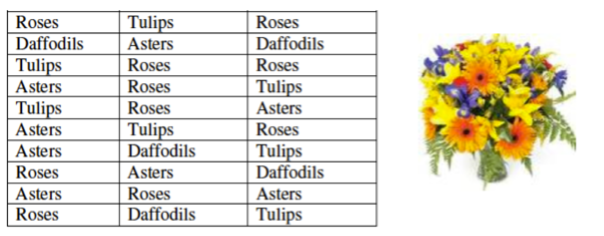Figure 1: Raw data from a survey of $\scriptsize 30$ ladies about their favourite flowersTable 1: Frequency table showing the favourite flowers of $\scriptsize 30$ ladies

In the case of the flowers, there were only four different types mentioned in the survey. In addition, the data was qualitative, so it was a simple matter of listing the four different flowers and then counting the frequency of each.

However, what if the data is quantitative, possibly continuous, and there are far more values in the data set like the data set below which represents the heights (in centimetres) of a group of $\scriptsize 90$ students.

$\scriptsize 165, 148, 158, 150, 160, 165, 150, 156, 155, 164,162, 160, 158, 148, 158, 140, 146, 160, 148, 152, 139, 165, 148, 160, 156, 158, 170, 155, 160,148, 155, 158, 179, 170, 158, 161, 155, 160, 163, 178, 138, 172, 170, 156, 160,160, 171, 140, 160, 170, 175, 148, 170, 177, 155, 167, 154, 160, 170, 155, 136, 179, 150, 167, 148, 160, 164, 167, 157, 165, 163, 140, 162, 178, 160, 170, 163, 162, 165, 175, 165, 152, 147, 180, 148, 170, 165, 167, 165$

Because the chances of any one height measured being reported more than once or twice is quite small, it would be silly to simply list all the values and count their frequency. In this case, we need to group the data into intervals or classes and then count how many values lie in each interval. Table 2 shows what the resulting frequency distribution for this raw data might look like.Table 2: Grouped data frequency table of the heights of $\scriptsize 90$ students### Take note!

The data in its raw form is called ungrouped data. It has not been organised in any way and is merely a list of the individual measurements. The data can be organised into intervals, and then presented in the form of a frequency distribution. We call this grouped data.

In Unit 3 of this Subject outcome, we will learn how to use this frequency distribution to create a histogram. For now, let’s focus on learning how to create a grouped frequency distribution.

Step 1:
The first step in creating a grouped frequency distribution is to work out what the range of the data is. Can you do this? What is the range of the raw data above?

Remember, to find the range we must subtract the smallest value from the largest value in the data set. This is $\scriptsize 180-136=44$.

Step 2:
The second step is to decide on the number of groups or classes you want. There are no hard and fast rules for this but, in general, you should not have fewer than five classes or more than $\scriptsize 15$ classes. You can experiment with the number of classes until you find one that you think fits the data best. Often, step 3 will help you decide on the number of classes to have. With a range of $\scriptsize 44$, about five or six classes probably makes sense.

Step 3:
In this step you find the class width; in other words, the difference between the lower class limit and the upper class limit. It is often a great idea to have an odd class width so that the midpoint of your class is a whole number. You will see why in Unit 3 when we draw histograms.

For now, if we were to have six classes, our class width would be $\scriptsize \displaystyle \frac{{\text{range}}}{{\text{classes}}}=\displaystyle \frac{{44}}{6}=7.33$. We always round up to the next whole number. So, the class width would be eight. This is not too bad, but it is not an odd number. Let’s try five classes.

Now our class width would be $\scriptsize \displaystyle \frac{{\text{range}}}{{\text{classes}}}=\displaystyle \frac{{44}}{5}=8.8$. Rounding up to the next whole number gives us a width of nine which is odd. Let’s go for five classes each with a width of nine.

Step 4:
Now it is time to create our frequency distribution table. The first column contains our class limits. The first class starts at the smallest value ($\scriptsize 136$) and we count up nine to get to the upper class limit. Therefore, the first upper class limit is $\scriptsize 144$ (see Table 3). This class will contain all the values from $\scriptsize 136$ up to $\scriptsize 144$.

The second lower class limit is $\scriptsize 145$ and we count up another nine to get to $\scriptsize 153$. Notice that there is a difference of nine (the class width) between each of the lower limits and upper class limits (see Table 3).

See if you can complete the class limits on your own before moving on to Step 5.

Table 4 shows all the class limits.

Step 5:
Now that we have our class limits, we can go through the data set and count how many values lie in each class. In Table 4 we have included a tally column to make this counting a little easier, but this is not strictly required, and we normally do not show frequency distributions with a tally column.

The first value in the data set is $\scriptsize 165$ so we put a tally mark in the $\scriptsize 163-171$ class row (see Table 5). The next value is $\scriptsize 148$ so we put a tally mark in the $\scriptsize 145-153$ class row. Continue like this through the rest of the values. To make counting the tally marks easier at the end, the fifth tally mark in a class is a cross through the previous four like this $\scriptsize \cancel{{|\ |\ |\ |}}$ or ||||.

See if you can complete the frequency distribution on your own before reading on.

### Did you know?

There is evidence of tally marks being used over $\scriptsize 20\ 000$ years ago. Tally sticks (see Figure 2) were also used thousands of years ago to aid counting and memory.

Table 6 shows the completed frequency distribution. Were you able to complete it correctly?

As noted above, we usually do not present the frequency distribution with any tally marks. Table 7 shows the final frequency distribution.

We can see, just by looking at the frequency of values in each interval, that the data follows a fairly normal distribution (most values are in the middle of the range). It is not significantly skewed positive or negative. This is not something we could ever have seen from the raw data.### Take note!

In the scenario above, all our values were rounded off to whole numbers. If you look at Table 7 again, you will see that the first class ends at $\scriptsize 144$ and the next class begins at $\scriptsize 145$. This was acceptable in this scenario because there were no fractional values that fell between any of the class limits.

However, most of the time with continuous data, this will not be the case and you will need to make sure that your classes are continuous, in other words that there are no gaps between them. The way to do this is to define your classes with inequalities as shown below.In this case, you would include all values equal to and bigger than $\scriptsize 136$ up to, but not including, $\scriptsize 145$ in the first interval.### Exercise 1.1

A group of learners counts the number of pairs of shoes each group member has. The data they collected is as follows:

$\scriptsize \{3,\ 6,\ 5,\ 14,\ 20,\ 4,\ 7,\ 14,\ 8,\ 5,\ 11,\ 19,\ 17,\ 16,\ 16,\ 13,\ 9,\ 6,\ 9,\ 13,\ 21,\ 7,\ 11,\ 9,\ 13,\ 5,\ 7\}$

Create a frequency distribution for the data using five intervals.

The full solutions are at the end of the unit.

## Stem and leaf plots

Another quick way to represent numeric or quantitative data is to use a stem and leaf plot. Stem and leaf plots, like frequency distributions, give you a quick way to see the ‘shape’ of the data. Is it normally distributed? Is it significantly negatively or positively skewed? Stem and leaf plots give you a way to summarise all the data in a set in one simple representation.

### Note

Stem and leaf plots are very quick and easy to create. Watch this video called “Stem & Leaf Plots” to find out how.

Stem & Leaf Plots (Duration: 02.53)### Example 1.1

Farmer Dlamini has a dairy with $\scriptsize 40$ cows. He recorded the quantity of milk (in litres) that each cow produced in a week in Table 8.

Draw a stem and leaf plot for this data.

Solution

Our stem will represent tens and the leaves will represent units. First list the stems.Now work through the data and add each value to the plot. The first value is $\scriptsize 41$ and is represented as follows:The second value is $\scriptsize 84$ and is represented as follows: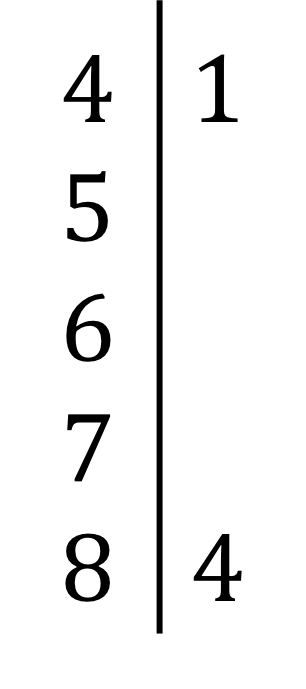Work through the rest of the values. Don’t forget to repeat duplicate values.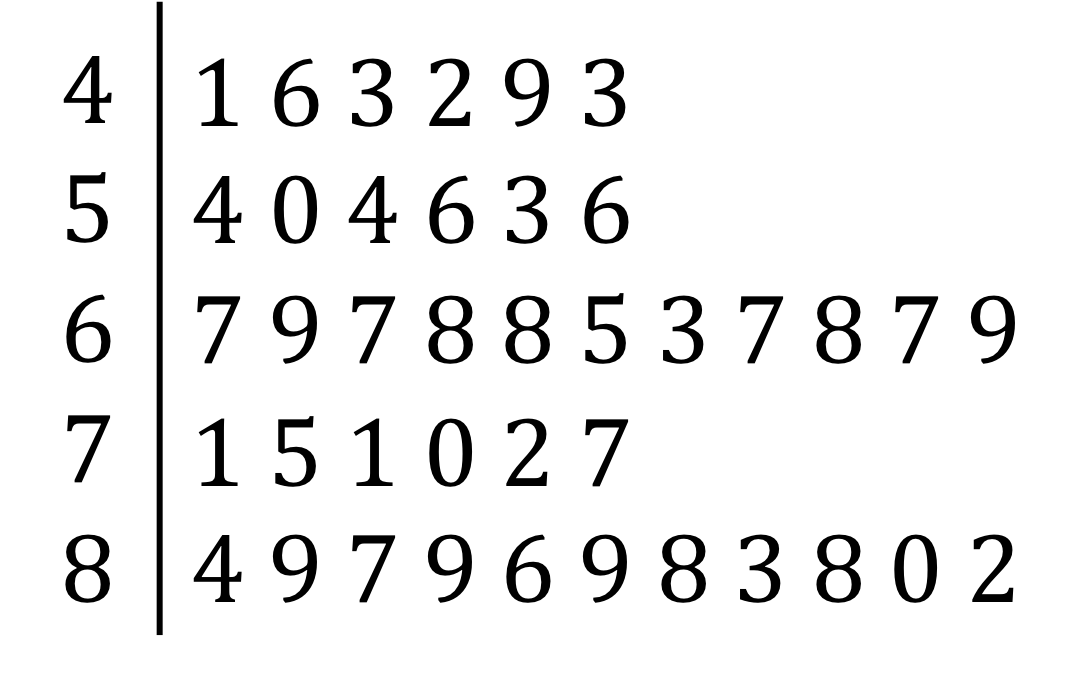Order the leaves in each stem from smallest to largest.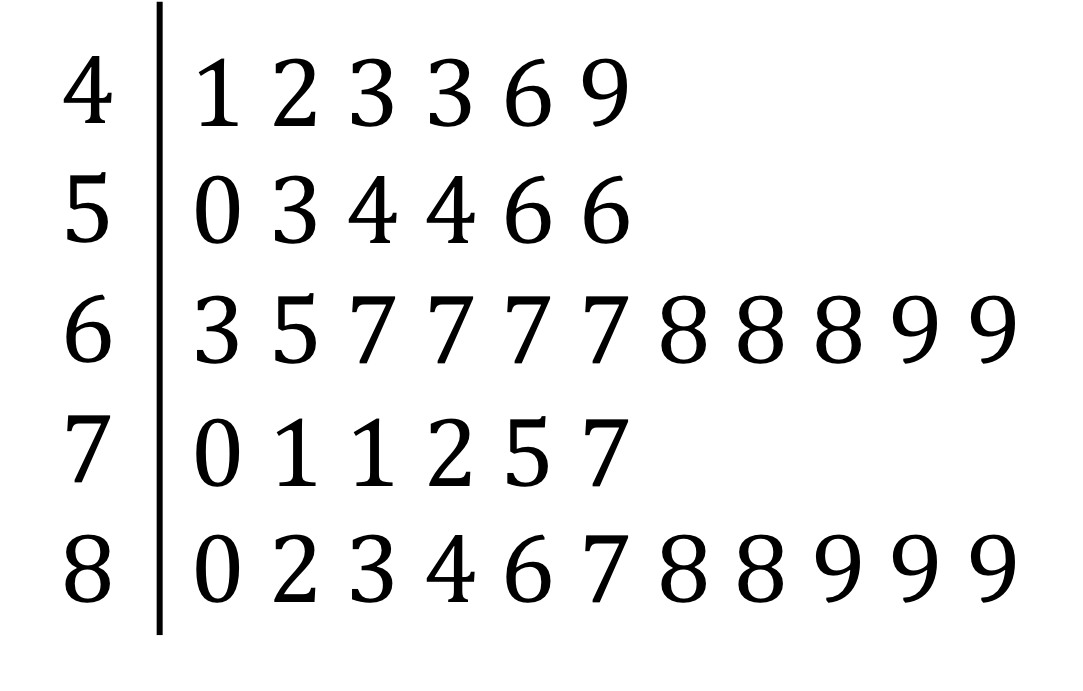### Exercise 1.2

Draw a stem and leaf plot of the following data.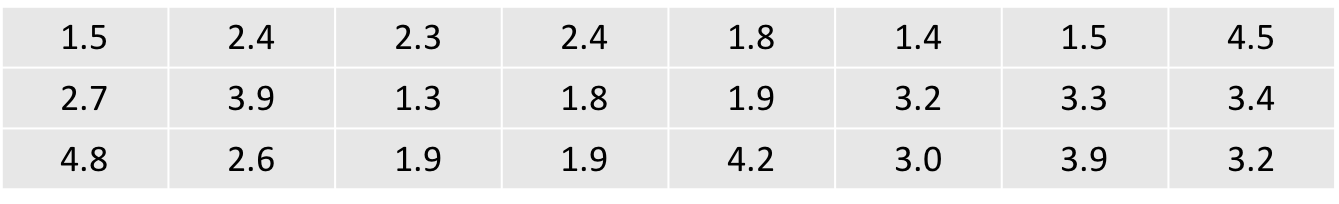The full solutions are at the end of the unit.

## Pie charts

Pie charts are a common way to represent data graphically. They are also easy to make with spreadsheet software like Microsoft Excel or Google Sheets. However, it is important that you can draw pie charts by hand with a protractor. Work through Activity 1.1 to learn how.### Activity 1.1: Draw a pie chart

Time required: 15 minutes

What you need:

• a pen or pencil
• a piece of paper
• a protractor

What to do:

The table below shows the total population of each part of the world. We are going to draw a pie chart to represent this data. Notice how the population data has been organised from greatest to smallest. When drawing a pie chart, it is always best to arrange your categories in descending order.1. Draw a circle on your piece of paper. It should have a radius of about $\scriptsize 10\ \text{cm}$. Now draw a line from the centre to the circumference straight up. This is where we will start measuring our pie slices from.
2. To calculate what proportion of the entire pie each region needs to occupy, we have to work out the total population first. Do this calculation.
3. Now work out the angle that you will need to draw to represent the Asia region by dividing Asia’s population by the total and then multiplying this proportion by $\scriptsize 360{}^\circ$. Round off to the nearest degree.
4. Measure this angle in a clockwise direction from the first line you drew and mark off this area to represent the Asia region.
5. Calculate the angles required to represent each of the other regions and measure and draw in these angles (in a clockwise direction), rounding off to the nearest degree.
6. Label each slice of your pie chart.
7. Calculate the total percentage of the total population in each region and add these figures below each label.
8. Give your pie chart a title. If you like, you can colour or shade in each slice to make them more distinct from each other.

What did you find?

1. .2. The total population is $\scriptsize 4\ 478+1\ 247+739+648+363+40=7\ 515$.
3. Asia angle: $\scriptsize \displaystyle \frac{{4\ 478}}{{7\ 515}}\times 360{}^\circ =215{}^\circ$
4. .5. Africa angle: $\scriptsize \displaystyle \frac{{1\text{ }247}}{{7\ 515}}\times 360{}^\circ =60{}^\circ$
Europe angle: $\scriptsize \displaystyle \frac{{739}}{{7\ 515}}\times 360{}^\circ =35{}^\circ$
Latin America and the Caribbean angle: $\scriptsize \displaystyle \frac{{648}}{{7\ 515}}\times 360{}^\circ =31{}^\circ$
North America angle: $\scriptsize \displaystyle \frac{{363}}{{7\ 515}}\times 360{}^\circ =17{}^\circ$
Oceania angle: $\scriptsize \displaystyle \frac{{40}}{{7\ 515}}\times 360{}^\circ =2{}^\circ$6. .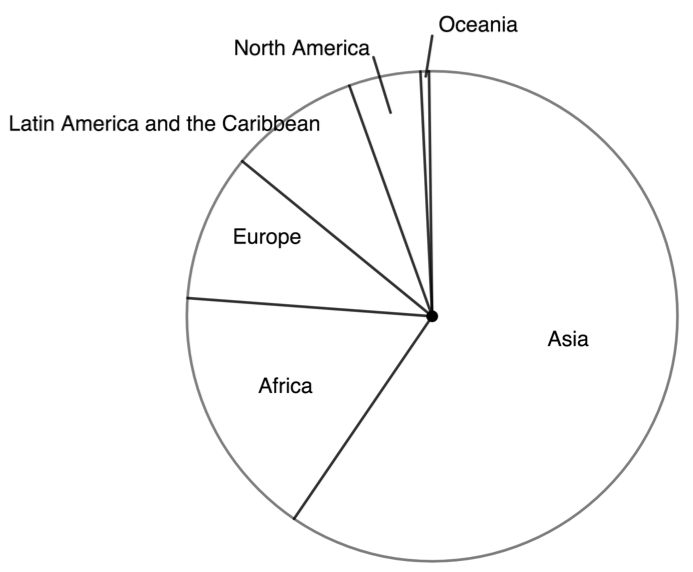7. .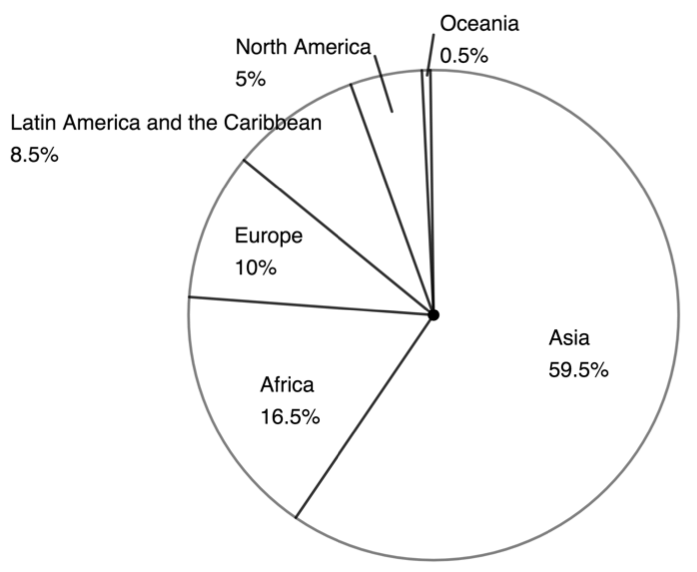8. .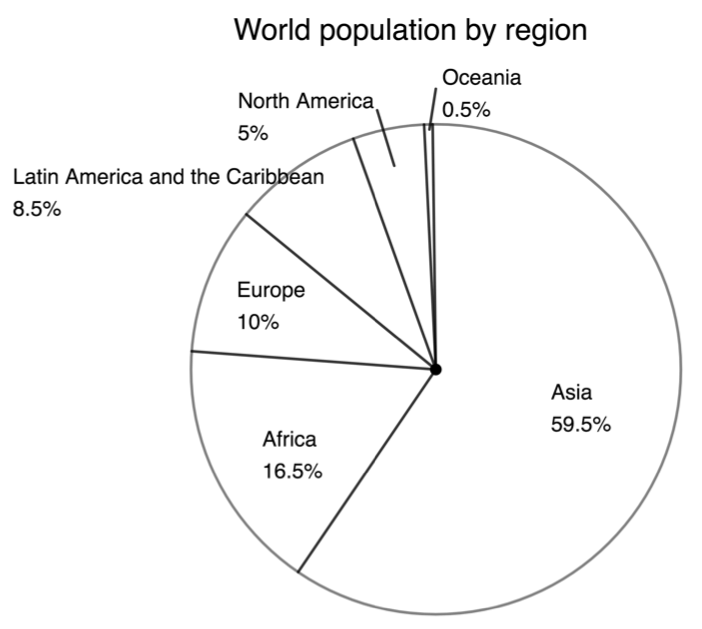### Note

If you still need more help in understanding how to complete this activity, watch the video called “Pie Charts” which works through this same example.

Pie Charts (Duration: 06.02)If you would like to watch another example of how to create a pie chart, watch this video called “Pie”

Pie Charts and Protractors (Duration: 05.06)### Exercise 1.3

Construct a pie chart of the following data. Show all your calculations. You may refer to the frequency distribution at the beginning of this unit to help you.The full solutions are at the end of the unit.

## Summary

In this unit you have learnt the following:

• How to create a grouped frequency distribution using intervals or classes to group numerical data.
• How to represent data using a stem and leaf plot.
• How to represent data using a pie chart.

# Unit 1: Assessment

#### Suggested time to complete: 40 minutes

Question 1 adapted from the NC(V) Mathematics Second Paper November 2012 Question 1.2.

1. GQ Magazine held a poll to determine the favourite DJs of NC(V) learners. The results of the first $\scriptsize 30$ responses are tabulated below.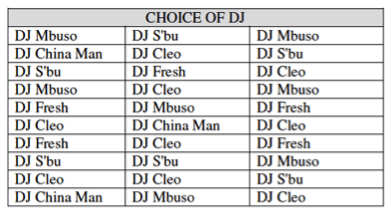Use the information in the table to answer the following questions.
1.  Complete the following frequency distribution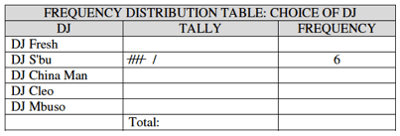2. Which DJ is most liked by these learners?
3. Use the completed table from question a. to construct a pie chart to represent the data.

Question 2 adapted from the NC(V) Mathematics Second Paper November 2012 Question 1.4.

1. The following data set shows the number of eggs laid by Mama Mchunu’s hens.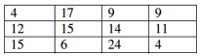Create a stem and leaf plot of the data.
2. A competition was held where students needed to guess the number of sweets in a jar. The closest guess would win the jar of sweets. The following guesses were recorded.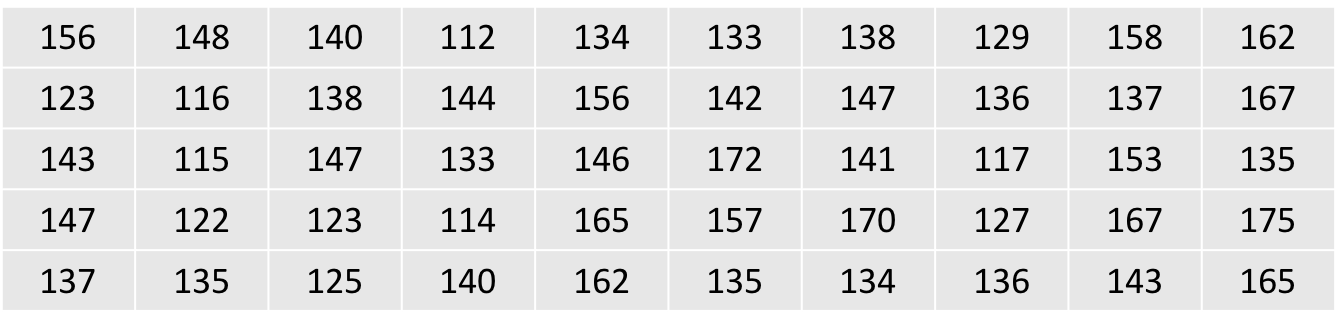Create a grouped frequency distribution for this data using a class width of $\scriptsize 10$.

The full solutions are at the end of the unit.

# Unit 1: Solutions

### Exercise 1.1

The range of the data is $\scriptsize 21-3=18$. We need to create five intervals or classes.
$\scriptsize \displaystyle \frac{{\text{range}}}{{\text{classes}}}=\displaystyle \frac{{18}}{5}=3.6$. Round this up to $\scriptsize 4$.Back to Exercise 1.1

### Exercise 1.2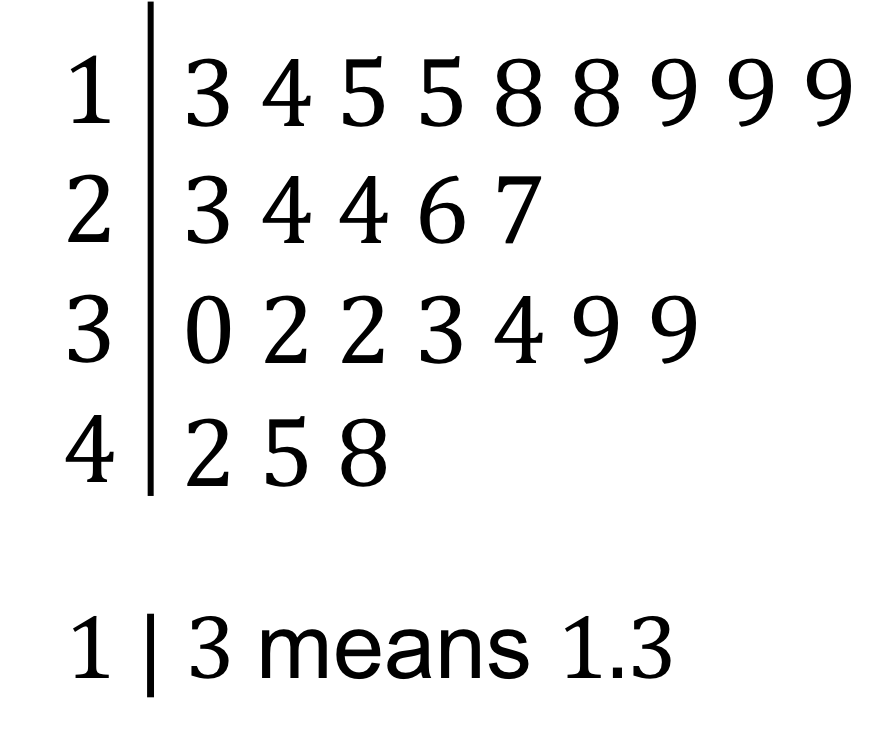Back to Exercise 1.2

### Exercise 1.3

The frequency distribution:Roses angle: $\scriptsize \displaystyle \frac{{10}}{{30}}\times 360{}^\circ =120{}^\circ$
Asters angle: $\scriptsize \displaystyle \frac{8}{{30}}\times 360{}^\circ =96{}^\circ$
Tulips angle: $\scriptsize \displaystyle \frac{7}{{30}}\times 360{}^\circ =84{}^\circ$
Daffodils angle: $\scriptsize \displaystyle \frac{5}{{30}}\times 360{}^\circ =60{}^\circ$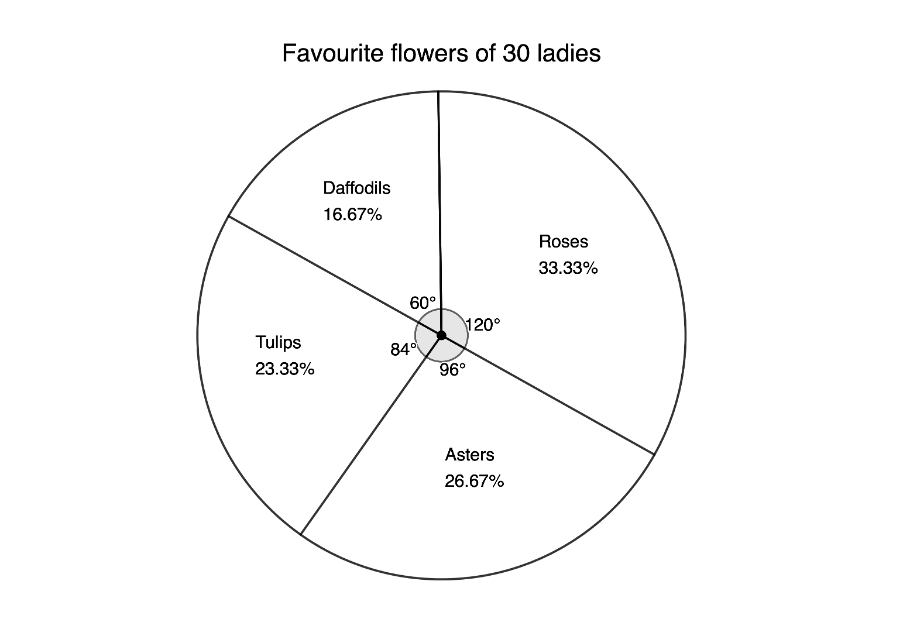Back to Exercise 1.3

### Unit 1: Assessment

1. .
1. .2. DJ Cleo is most liked.
3. DJ Fresh: $\scriptsize \displaystyle \frac{5}{{30}}\times 360{}^\circ =60{}^\circ$
DJ S’bu: $\scriptsize \displaystyle \frac{6}{{30}}\times 360{}^\circ =72{}^\circ$
DJ China Man: $\scriptsize \displaystyle \frac{3}{{30}}\times 360{}^\circ =36{}^\circ$
DJ Cleo: $\scriptsize \displaystyle \frac{9}{{30}}\times 360{}^\circ =108{}^\circ$
DJ Mabuso: $\scriptsize \displaystyle \frac{7}{{30}}\times 360{}^\circ =84{}^\circ$2. .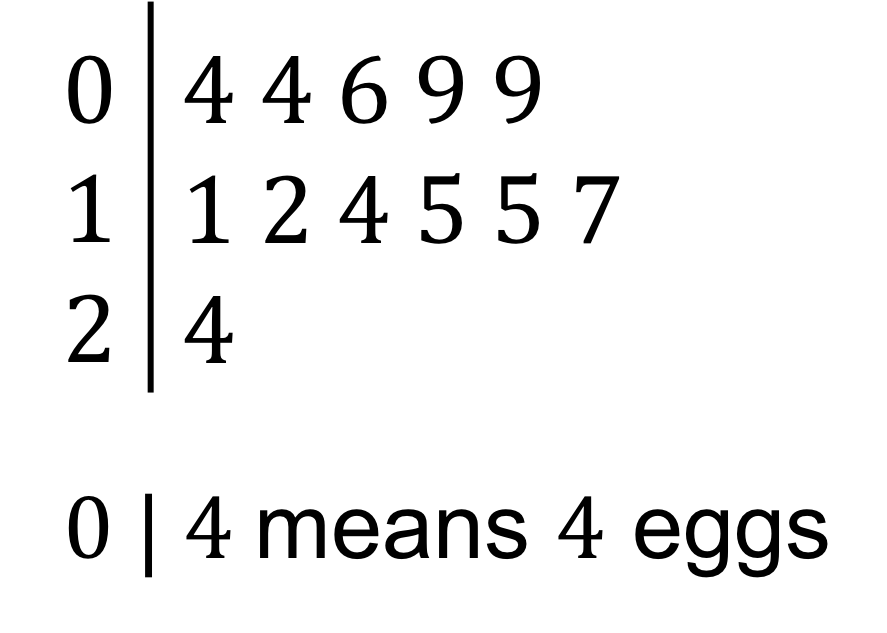3. The class width must be $\scriptsize 10$. The lowest value is $\scriptsize 112$. Therefore, the first class will be $\scriptsize 112\le x \lt 122$.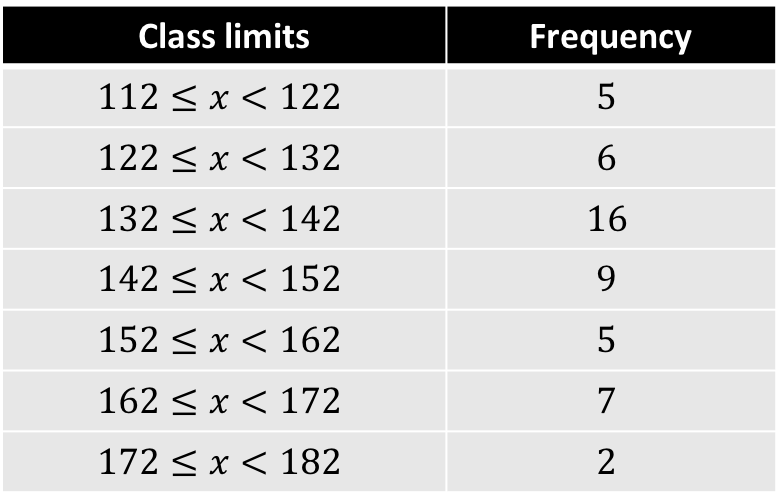Back to Unit 1: Assessment